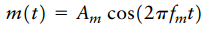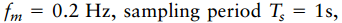Create an Account

Home / Questions / a Plot the spectrum of a PAM wave produced by the modulating signal assuming the...

a Plot the spectrum of a PAM wave produced by the modulating signal assuming the modulation frequency and pulse duration

(a) Plot the spectrum of a PAM wave produced by the modulating signalassuming the modulation frequencyand pulse duration

T = 0.45s

(b) Using an ideal reconstruction filter, plot the spectrum of the filter output. Compare this result with the output that would be obtained if there were no aperture effect.

Jul 31 2020 View more View LessSubscribe To Get Solution